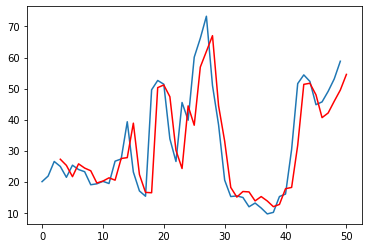Course Content

# Time Series Analysis

1. Time Series: Let's Start

2. Time Series Processing

3. Time Series Visualization

Time Series Analysis

##Autoregression

Let's move on to the review of the autoregressive model:The formula is similar to the linear regression formula, which is where the name comes from. Instead of the coefficient - the past value of `x` is used.

With `statsmodels` we can run an autoregressive model `AutoReg()`:If you notice, the predictions made by the autoregressive model are more accurate than those of the simple moving average.

Let's learn how to evaluate the received results of the trained models. The error is calculated using the mean-squared error. This is done simply with the help of functions `sqrt()` and `mean_squared_error()`:

Test MSE: 2.072

In the same way, we calculate the error value for the previous model:

Test MSE: 5.321

The smaller the MSE value, the correspondingly smaller the error.

Create an autoregressive model and train it on the dataset `shampoo.csv`.
1. Create an autoregression model (`Autoreg`) with 6 lags for the `"Sales"` column of the `df` DataFrame.
2. Fit the `model` to data.
3. Make predictions using the `model`. Start forecasting at the first row (the `start` parameter), and set the `dynamic` parameter to `False`.
4. Visualize the results: show the first 150 observations of the `"Sales"` column of the `df` DataFrame within the first call of the `.plot()` function and the first 150 predicted values within the second call.